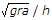Last updated: August 14, 2020

1. Work done is said to be zero, when
2. The distance, between the point of projection and the point where the projectile strikes the ground, is known as range.
3. When a rigid body is suspended vertically, and it oscillates with a small amplitude under the action of the force of gravity, the body is known as
4. The angle between two forces when the resultant is maximum and minimum respectively are
5. One watt is equal to
6. The algebraic sum of the resolved parts of a number of forces in a given direction is equal to the resolved part of their resultant in the same direction. This is known as
7. The equivalent length of a simple pendulum which gives the same frequency as compound pendulum is
8. During elastic impact, the relative velocity of the two bodies after impact is __________ the relative velocity of the two bodies before impact.
9. Mass moment of inertia of a uni form thin rod of mass M and length (l) about its mid-point and perpendicular to its length is
10. If two blocks of equal mass are attached to the two ends of a light string and one of the blocks is placed over a smooth horizontal plane while the other is hung freely after passing over a smooth pulley, then the two blocks will have some motion.
11. The resultant of two forces P and Q acting at an angle θ is
12. A weight of 1000 N can be lifted by an effort of 80 N. If the velocity ratio is 20, the machine is
13. The rate of change of momentum is directly proportional to the impressed force, and takes place in the same direction in which the force acts. This statement is known as
14. If a number of forces acting at a point be represented in magnitude and direction by the three sides of a triangle, taken in order, then the forces are in equilibrium.
15. Which of the following are vector quantities?
16. The angular velocity (in rad / s) of a body rotating at N revolutions per minute is
17. Newton's second law motion __________ a relation between force and mass of a moving body.
18. The overturning of a vehicle on a level circular path can be avoided if the velocity of vehicle is __________19. The minimum force required to slide a body of weight W on a rough horizontal plane is
20. The maximum acceleration of a particle moving with simple harmonic motion is
Engineering Mechanics MCQ 3
You got {{userScore}} out of {{maxScore}} correct
{{title}}
{{image}}
{{content}}